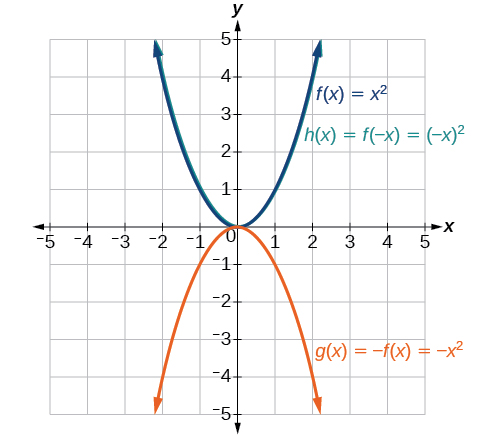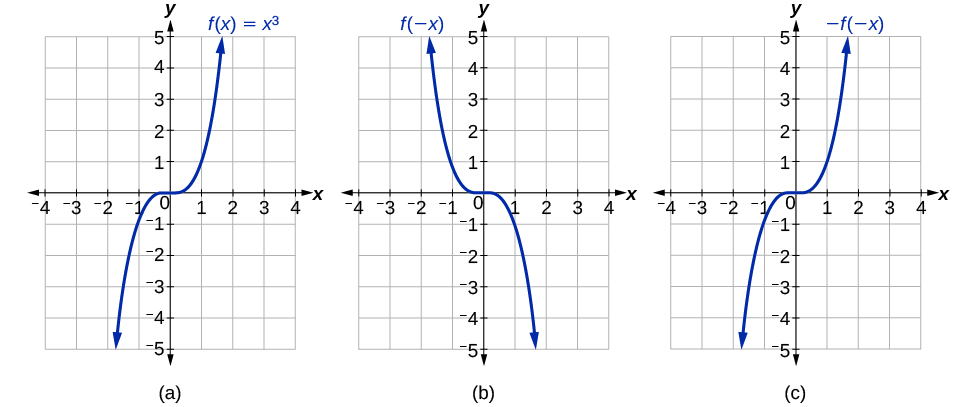# 1.5 Transformation of functions  (Page 6/22)

 Page 6 / 22

Given the toolkit function $\text{\hspace{0.17em}}f\left(x\right)={x}^{2},\text{\hspace{0.17em}}$ graph $\text{\hspace{0.17em}}g\left(x\right)=-f\left(x\right)\text{\hspace{0.17em}}$ and $\text{\hspace{0.17em}}h\left(x\right)=f\left(-x\right).\text{\hspace{0.17em}}$ Take note of any surprising behavior for these functions.Notice: $\text{\hspace{0.17em}}g\left(x\right)=f\left(-x\right)\text{\hspace{0.17em}}$ looks the same as $\text{\hspace{0.17em}}f\left(x\right)$ .

## Determining even and odd functions

Some functions exhibit symmetry so that reflections result in the original graph. For example, horizontally reflecting the toolkit functions $f\left(x\right)={x}^{2}$ or $f\left(x\right)=|x|$ will result in the original graph. We say that these types of graphs are symmetric about the y -axis. Functions whose graphs are symmetric about the y -axis are called even functions.

If the graphs of $\text{\hspace{0.17em}}f\left(x\right)={x}^{3}\text{\hspace{0.17em}}$ or $\text{\hspace{0.17em}}f\left(x\right)=\frac{1}{x}\text{\hspace{0.17em}}$ were reflected over both axes, the result would be the original graph, as shown in [link] .(a) The cubic toolkit function (b) Horizontal reflection of the cubic toolkit function (c) Horizontal and vertical reflections reproduce the original cubic function.

We say that these graphs are symmetric about the origin. A function with a graph that is symmetric about the origin is called an odd function .

Note: A function can be neither even nor odd if it does not exhibit either symmetry. For example, $\text{\hspace{0.17em}}f\left(x\right)={2}^{x}\text{\hspace{0.17em}}$ is neither even nor odd. Also, the only function that is both even and odd is the constant function $\text{\hspace{0.17em}}f\left(x\right)=0.$

## Even and odd functions

A function is called an even function    if for every input $\text{\hspace{0.17em}}x$

$f\left(x\right)=f\left(-x\right)$

The graph of an even function is symmetric about the $y\text{-}$ axis.

A function is called an odd function    if for every input $\text{\hspace{0.17em}}x$

$f\left(x\right)=-f\left(-x\right)$

The graph of an odd function is symmetric about the origin.

Given the formula for a function, determine if the function is even, odd, or neither.

1. Determine whether the function satisfies $\text{\hspace{0.17em}}f\left(x\right)=f\left(-x\right).\text{\hspace{0.17em}}$ If it does, it is even.
2. Determine whether the function satisfies $\text{\hspace{0.17em}}f\left(x\right)=-f\left(-x\right).\text{\hspace{0.17em}}$ If it does, it is odd.
3. If the function does not satisfy either rule, it is neither even nor odd.

## Determining whether a function is even, odd, or neither

Is the function $\text{\hspace{0.17em}}f\left(x\right)={x}^{3}+2x\text{\hspace{0.17em}}$ even, odd, or neither?

Without looking at a graph, we can determine whether the function is even or odd by finding formulas for the reflections and determining if they return us to the original function. Let’s begin with the rule for even functions.

$f\left(-x\right)={\left(-x\right)}^{3}+2\left(-x\right)=-{x}^{3}-2x$

This does not return us to the original function, so this function is not even. We can now test the rule for odd functions.

$-f\left(-x\right)=-\left(-{x}^{3}-2x\right)={x}^{3}+2x$

Because $\text{\hspace{0.17em}}-f\left(-x\right)=f\left(x\right),\text{\hspace{0.17em}}$ this is an odd function.

Is the function $\text{\hspace{0.17em}}f\left(s\right)={s}^{4}+3{s}^{2}+7\text{\hspace{0.17em}}$ even, odd, or neither?

even

## Graphing functions using stretches and compressions

Adding a constant to the inputs or outputs of a function changed the position of a graph with respect to the axes, but it did not affect the shape of a graph. We now explore the effects of multiplying the inputs or outputs by some quantity.

We can transform the inside (input values) of a function or we can transform the outside (output values) of a function. Each change has a specific effect that can be seen graphically.

## Vertical stretches and compressions

When we multiply a function by a positive constant, we get a function whose graph is stretched or compressed vertically in relation to the graph of the original function. If the constant is greater than 1, we get a vertical stretch ; if the constant is between 0 and 1, we get a vertical compression . [link] shows a function multiplied by constant factors 2 and 0.5 and the resulting vertical stretch and compression.

a colony of bacteria is growing exponentially doubling in size every 100 minutes. how much minutes will it take for the colony of bacteria to triple in size
what is the importance knowing the graph of circular functions?
can get some help basic precalculus
What do you need help with?
Andrew
how to convert general to standard form with not perfect trinomial
can get some help inverse function
ismail
Rectangle coordinate
how to find for x
it depends on the equation
Robert
whats a domain
The domain of a function is the set of all input on which the function is defined. For example all real numbers are the Domain of any Polynomial function.
Spiro
foci (–7,–17) and (–7,17), the absolute value of the differenceof the distances of any point from the foci is 24.
difference between calculus and pre calculus?
give me an example of a problem so that I can practice answering
x³+y³+z³=42
Robert
dont forget the cube in each variable ;)
Robert
of she solves that, well ... then she has a lot of computational force under her command ....
Walter
what is a function?
I want to learn about the law of exponent
explain this
what is functions?
A mathematical relation such that every input has only one out.
Spiro
yes..it is a relationo of orders pairs of sets one or more input that leads to a exactly one output.
Mubita
Is a rule that assigns to each element X in a set A exactly one element, called F(x), in a set B.
RichieRich
If the plane intersects the cone (either above or below) horizontally, what figure will be created?ByByBy Brooke DelaneyBy OpenStaxBy Yasser IbrahimBy MldelatteBy Sarah WarrenBy OpenStaxBy Heather McAvoyByBy OpenStaxBy Mistry Bhavesh